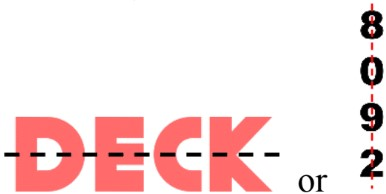# Basics of symmetry#### Everything You Need in One Place

Homework problems? Exam preparation? Trying to grasp a concept or just brushing up the basics? Our extensive help & practice library have got you covered.#### Learn and Practice With Ease

Our proven video lessons ease you through problems quickly, and you get tonnes of friendly practice on questions that trip students up on tests and finals.#### Instant and Unlimited Help

Our personalized learning platform enables you to instantly find the exact walkthrough to your specific type of question. Activate unlimited help now!

0/4
##### Intros
###### Lessons
1. Introduction to Basics of Symmetry:
2. What is symmetry? What is a symmetrical shape?
3. Folding test for lines of symmetry
4. Mirror test for lines of symmetry
5. Symmetry in alphabet letters and numbers
0/19
##### Examples
###### Lessons
1. Congruent parts and lines of symmetry
A dotted line breaks the shape into two parts.
• Is it a line of symmetry?
• Are the parts congruent?
1.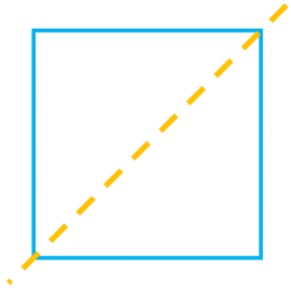2.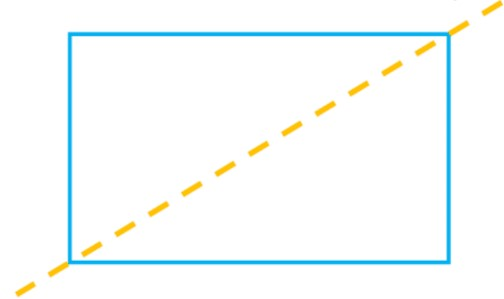3.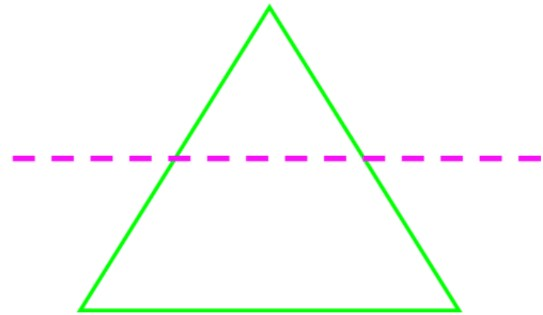4.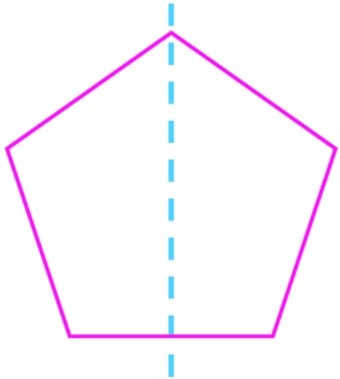2. Line of symmetry
Draw a line of symmetry for the shape.
1.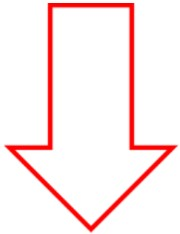2.3.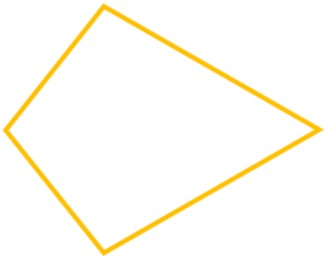4.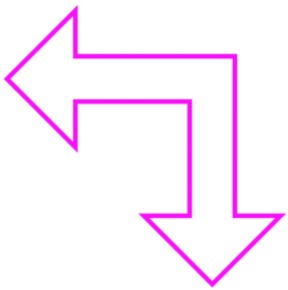5.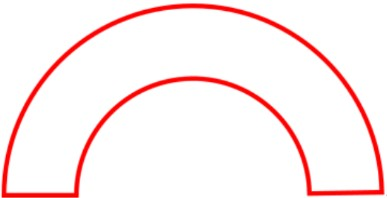6.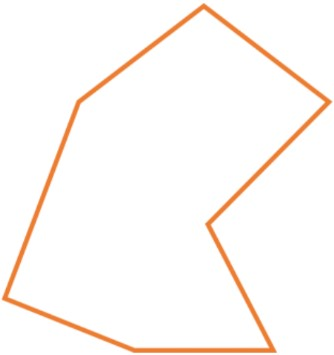3. Multiple lines of symmetry
Draw two possible lines of symmetry.
1.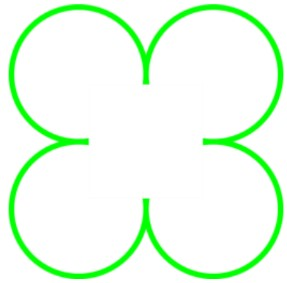2.3.4. Symmetrical shapes on a dot grid
Using the dot grid, finish drawing the shape. The line of symmetry and half of the shape are shown.
1.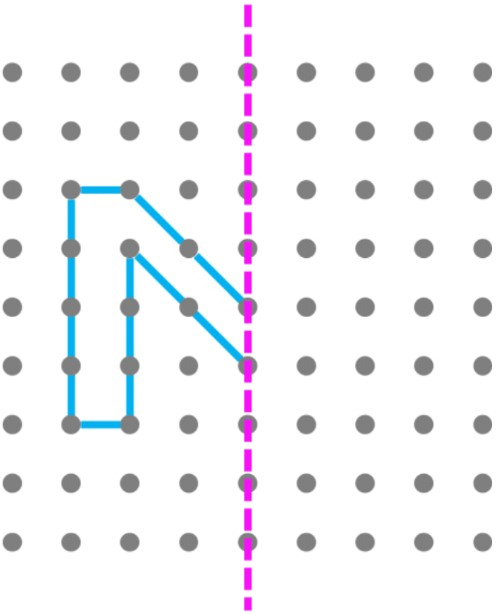2.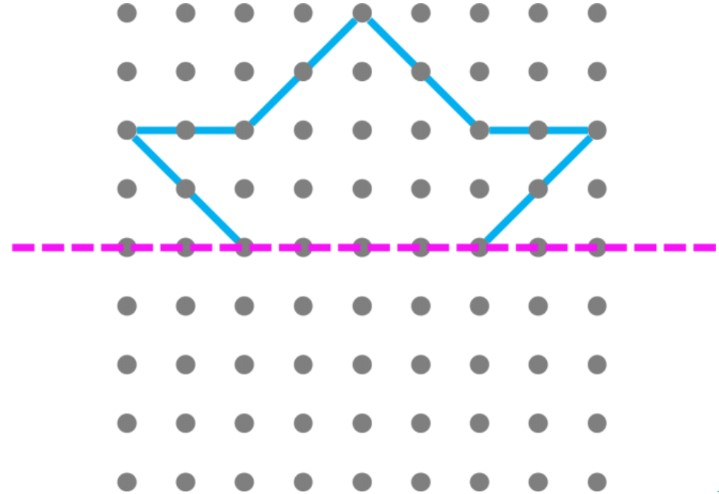3.5. Symmetry of regular polygons
1. For each regular polygon, draw all possible lines of symmetry and fill out your answers in the table.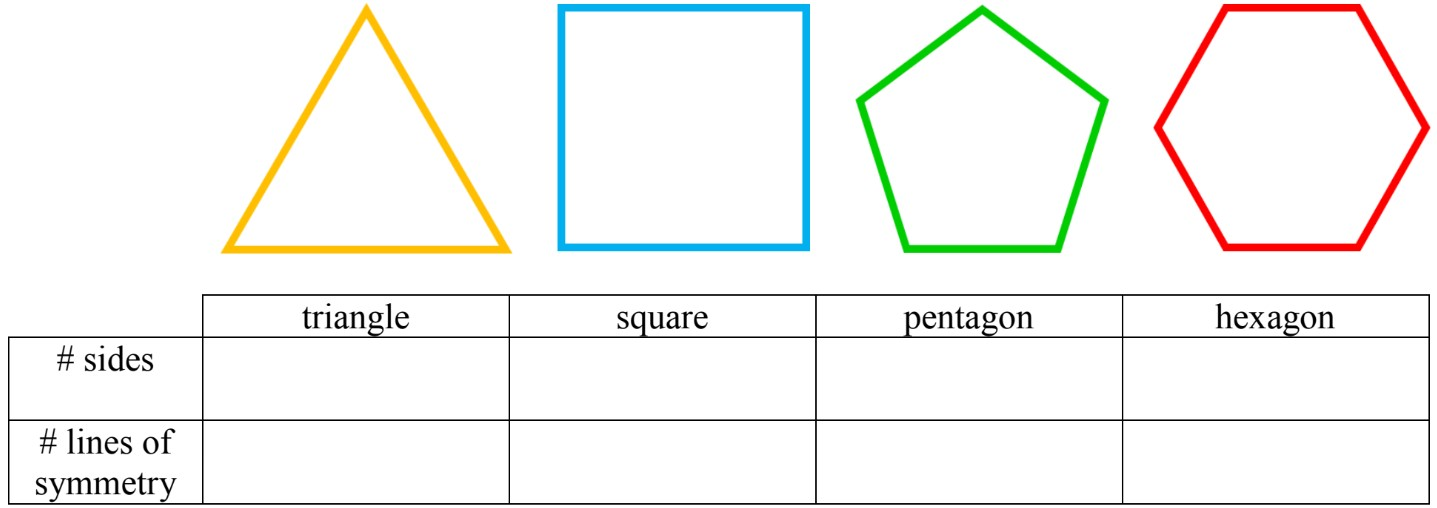2. Following the pattern for regular polygons, how many lines of symmetry would a heptagon have?
3. Following the pattern for regular polygons, how many lines of symmetry would an octagon have?
0%
##### Practice
###### Topic Notes

In this lesson, we will learn:

• How to identify symmetrical vs. asymmetrical shapes or pictures
• How to check symmetry lines with the folding method or mirror test method

Notes:

• A "symmetrical" shape is made up of exact same parts facing each other
• A line of symmetry divides a symmetrical shape into 2 congruent parts
• If the shape is not symmetrical it is "asymmetrical"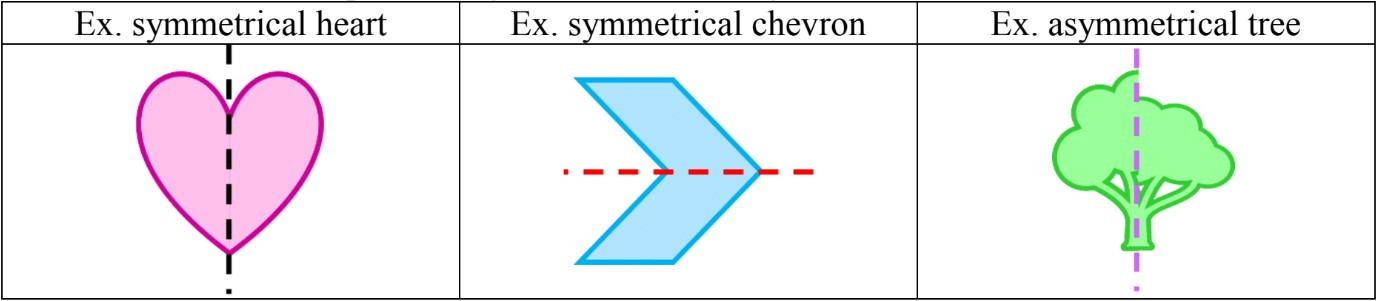• Check if a shape has a line of symmetry by folding it in half to see if the parts match up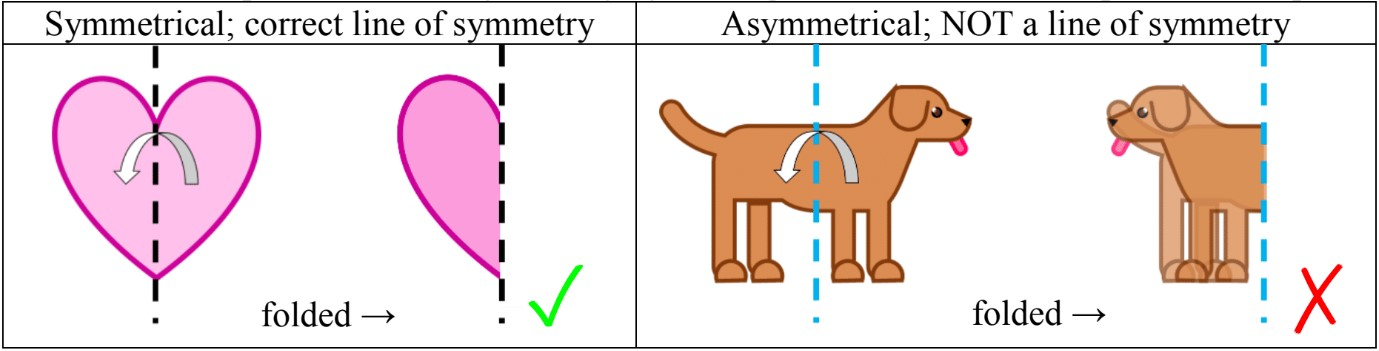• or using a mirror test: laying the picture/shape flat and putting a mirror on a possible symmetry line to see if the mirrored image matches with the original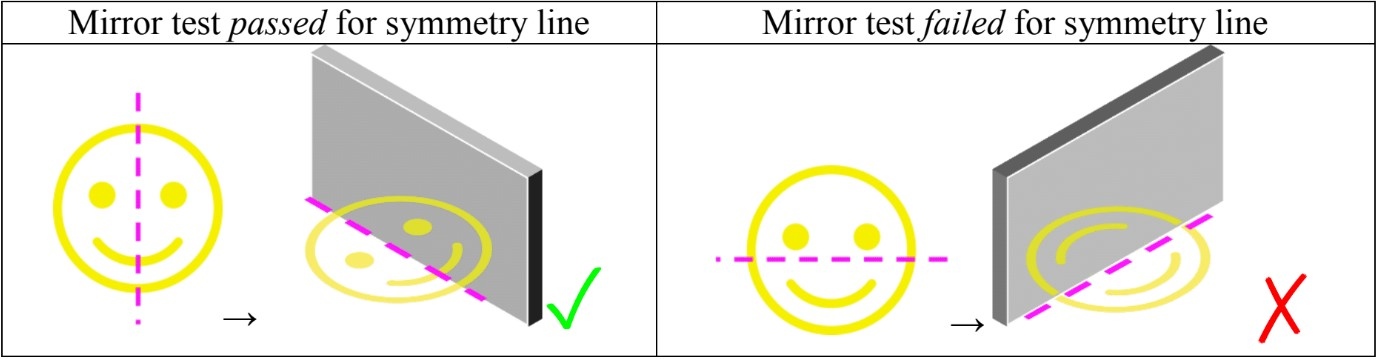• Some shapes have more than one line of symmetry. Regular polygons have the same number of symmetry lines as number of sides

• Ex. triangles = 3 sides = 3 symmetry lines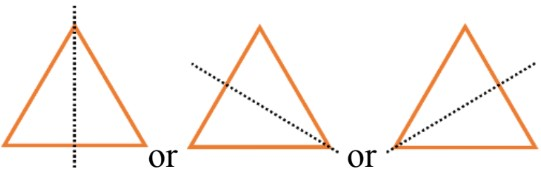• some words (alphabet letters) and numbers also have symmetry: